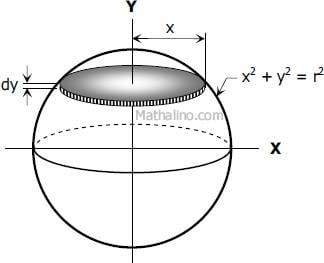# Using infinitesimals to find the volume of a sphere/surface

• B
• EddiePhys
In summary, the author is trying to find the surface area of a sphere using single variable calculus, but gets confused because the surface is not orthogonal to the y-direction.f

#### EddiePhys

I've always thought of dxat the end of an integral as a "full stop" or something to tell me what variable I'm integrating with respect to.
I looked up the derivation of the formula for volume of a sphere, and here, dx is taken as an infinitesimally small change which is multiplied by the area of a disc(pi r^2) giving $$\displaystyle V = 2\pi \int_0^r x^2 dy$$ which is the sum of these infinitesimals.Now I'm really confused. Is it correct to think of it this way? Is there any other way to prove this result without using infinitesimals? Also, if I'm integrating from 0 to r, wouldn't this give me the area of only half the sphere?

Part two to my question:
Using this same logic of using infinitesimals, I tried to find the surface area of a sphere and looked at it as the sum of infinite rings.

$$\displaystyle A = \int_0^r 2\pi x dy$$
$$=> \displaystyle A = \int_0^r 2\pi \sqrt{r^2-y^2} dy$$
But this is wrong. Why?

But this is wrong. Why?
You are failing to account for the fact that the surface element is not orthogonal to the y-direction.

Also, if I'm integrating from 0 to r, wouldn't this give me the area of only half the sphere?
Yes, but you have multiplied your integral by 2, so it's okay. Or you can omit the 2 and integrate from -r to +r. Same thing

You are failing to account for the fact that the surface element is not orthogonal to the y-direction.

I'm sorry, I don't understand. How is this any different from the volume case?

I'm sorry, I don't understand. How is this any different from the volume case?
Draw a picture of your circle. At a given y, draw a section of the circle of width dy. That section is a straight rod (a supposed to the disk that you had in the case of the sphere). The analogue of the volume of the disk that you had for the case of the sphere (which was ##\pi dy x^2##) is now the area of the of the rod, which is width x length = dy times 2 x, where ## x = \sqrt{R^2-y^2} ##. Now integrate over y from 0 to R (and then multiply by 2 to get the total area of the circle).

Surface area when using single variable calculus without parametrization requires the use of Lagrange's mean value theorem. So, instead of using dy, there is a need to use the infinitesimal ds.

Try deriving the inertia of an inverted isosceles triangle using single variable calculus.

Last edited:
For your surface area equation, what you're doing is constructing cylinders, and the integral will add all of the surface areas of the cylinders to get the total surface area. The problem is that when you construct cylinders, like the one in the picture, the endpoints of the cylinder do not trace the surface of the circle, so your integral will not be accurate. Notice in the picture, the top-right of the cylinder touches the circle's surface, but the bottom-right of the cylinder is not actually touching the circle, it is off by a bit.

You should try finding the surface integral using two parameters theta and z. Try taking the cross product to find the vector that points in direction of gradient. It's that easy